Function Repository Resource:

# BitFlip

Flip an individual bit in an integer (0 to 1 and 1 to 0)

Contributed by: Arnoud Buzing
 ResourceFunction["BitFlip"][n,p] flips the pth bit of integer n.

## Details and Options

The lowest bit of integer n (e.g. signifying 20) is flipped with p=0.

## Examples

### Basic Examples (3)

Flip the 0th bit of the number 2 from a 0 to a 1:

 In:=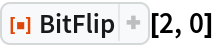Out=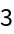Flip the 0th bit of the number 3 from a 1 to a 0:

 In:=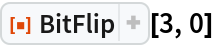Out=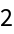Starting with the number 0, flip positional bits:

 In:=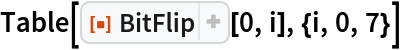Out=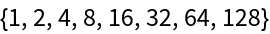### Scope (2)

Flip the bit associated with 24:

 In:=Out=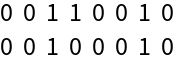Flip the bit associated with 27:

 In:=Out=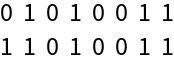### Applications (1)

Compute the Hamming distance between a random integer and the same number with a single random bit flipped:

 In:=Out=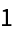Arnoud Buzing

## Requirements

Wolfram Language 11.3 (March 2018) or above

## Version History

• 1.0.0 – 29 January 2019